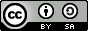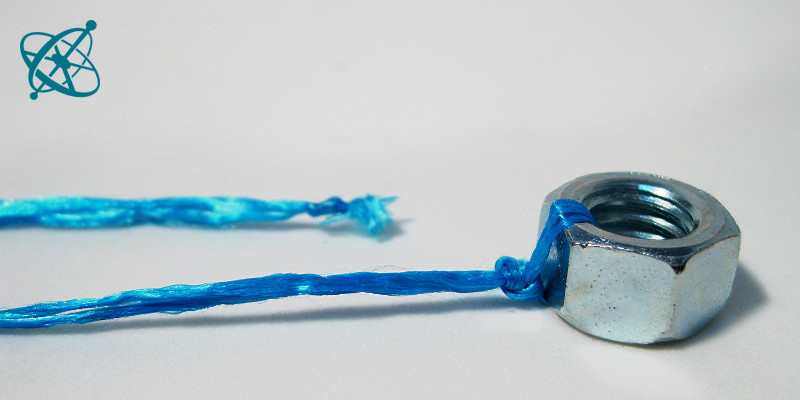•What type of energy makes a pendulum swing?

•www.sciensation.org | Ciênsação hands-on experiments are published as Open Educational resources under a Creative Commons Attribution-ShareAlike 4.0 International License.Physics
age: 12 – 16

# To and fro kinetic and potential energy

A pendulum is a good example of how energy can be transformed from one type to another: As it swings back and forth, there is a constant transfer between kinetic energy and gravitational potential energy.

Learning objective

A pendulum exchanges potential for kinetic energy, and vice versa, while the total energy remains the same.

At its highest point, a pendulum has potential energy, at its lowest point, kinetic energy.

String
Weight, e.g. metallic nut

Make a simple pendulum from a string and a metallic nut as weight.

1. What kind of energy makes a pendulum swing?

2. Is this energy constant?

Guiding Questions (if needed)

Does a pendulum have kinetic energy?
› Yes. You can start a pendulum if you add kinetic energy by pushing its weight to the side.

Does a pendulum have potential energy?
› Yes. You can slow down or accelerate a pendulum by lowering or lifting the pivot, thus withdrawing or adding potential energy.

Does it always have these two forms of energy simultaneously?
› No. At its highest point it has no kinetic energy. One can just freeze its motion there, setting the kinetic energy to zero, and release it at any time later, without the pendulum loosing any energy. At the lowest point, however, all potential energy is transformed in kinetic energy: stopping the motion there makes the pendulum loose all its energy.

Does the total energy change?
› No. While the type of energy changes, the total energy remains constant – except for a tiny bit of friction that ultimately will stop the swinging.

Findings

A pendulum has not a single type of energy but exchanges kinetic for potential energy and back. As long as the system is undisturbed, the total energy remains the same. You can observe the effect of adding or withdrawing these two types of energy to the system by lifting or lowering it during the movement (potential energy) or by stopping or accelerating it at the bottom (kinetic energy).

Related Experiments